The perfect place for easy learning...

×

# Python Scope

The scope refers to the accessibility of a variable or object in the program. The scope of a variable determines the part of the program in which it can be accessed or used. In simple terms, the scope of a variable is the region of the program in which that variable can access.

In Python programming, there are four different levels of scope and they are as follows.

• Local Scope
• Enclosed Scope
• Global Scope
• Built-in Scope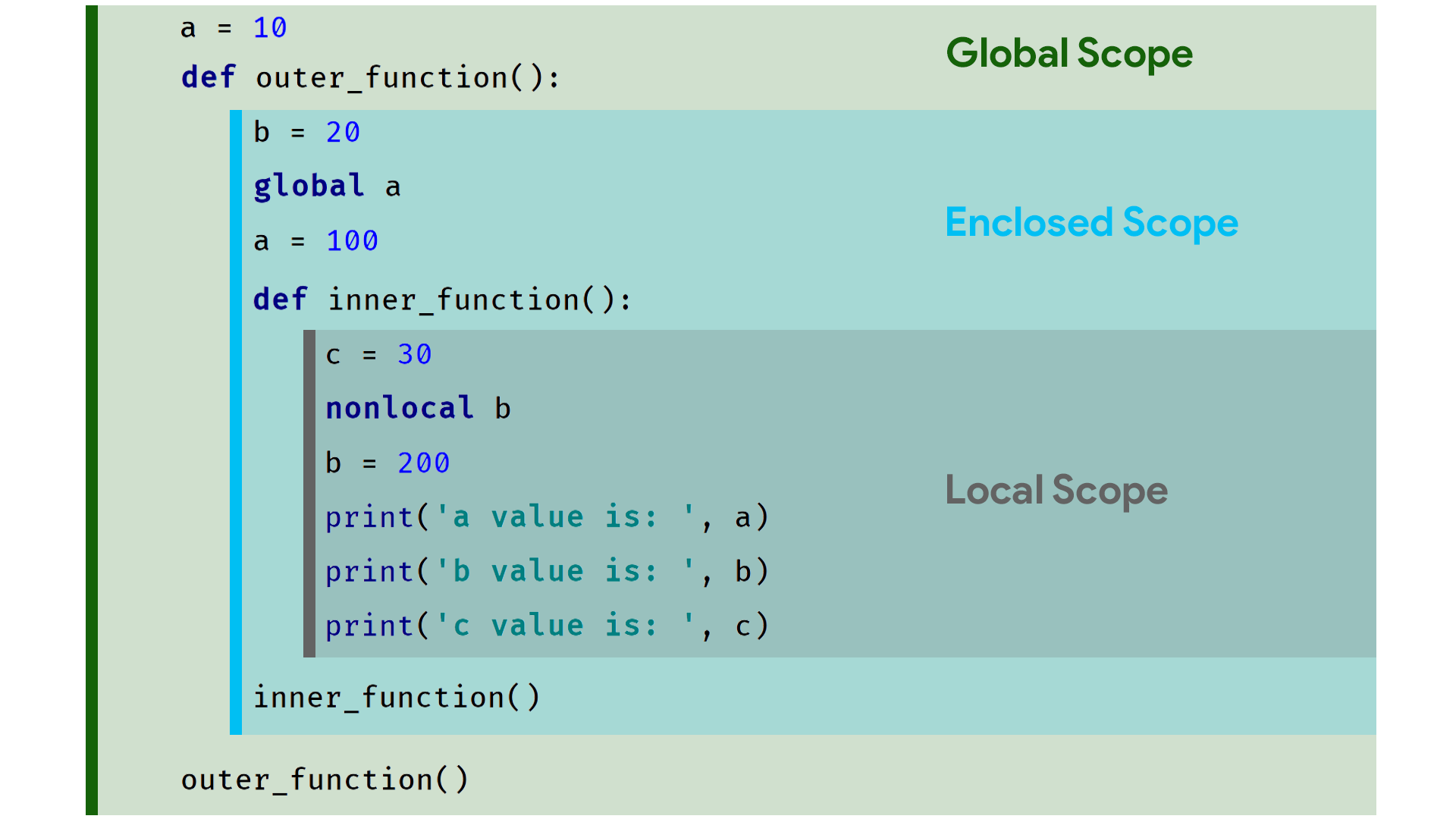# Local Scope

In Python, the variable that created inside a function is said to be under the local scope. The variable with a local scope has its visibility inside the function in which it has created. That means the variable with the local scope is accessible only inside the function in which it has created.

Example
``````def my_function():
a = 10
print('Inside my_function a value is: ', a)

my_function()
#print('Outside my_function a value is: ', a)    # Not accessible
``````

When we run the above example code, it produces the following output.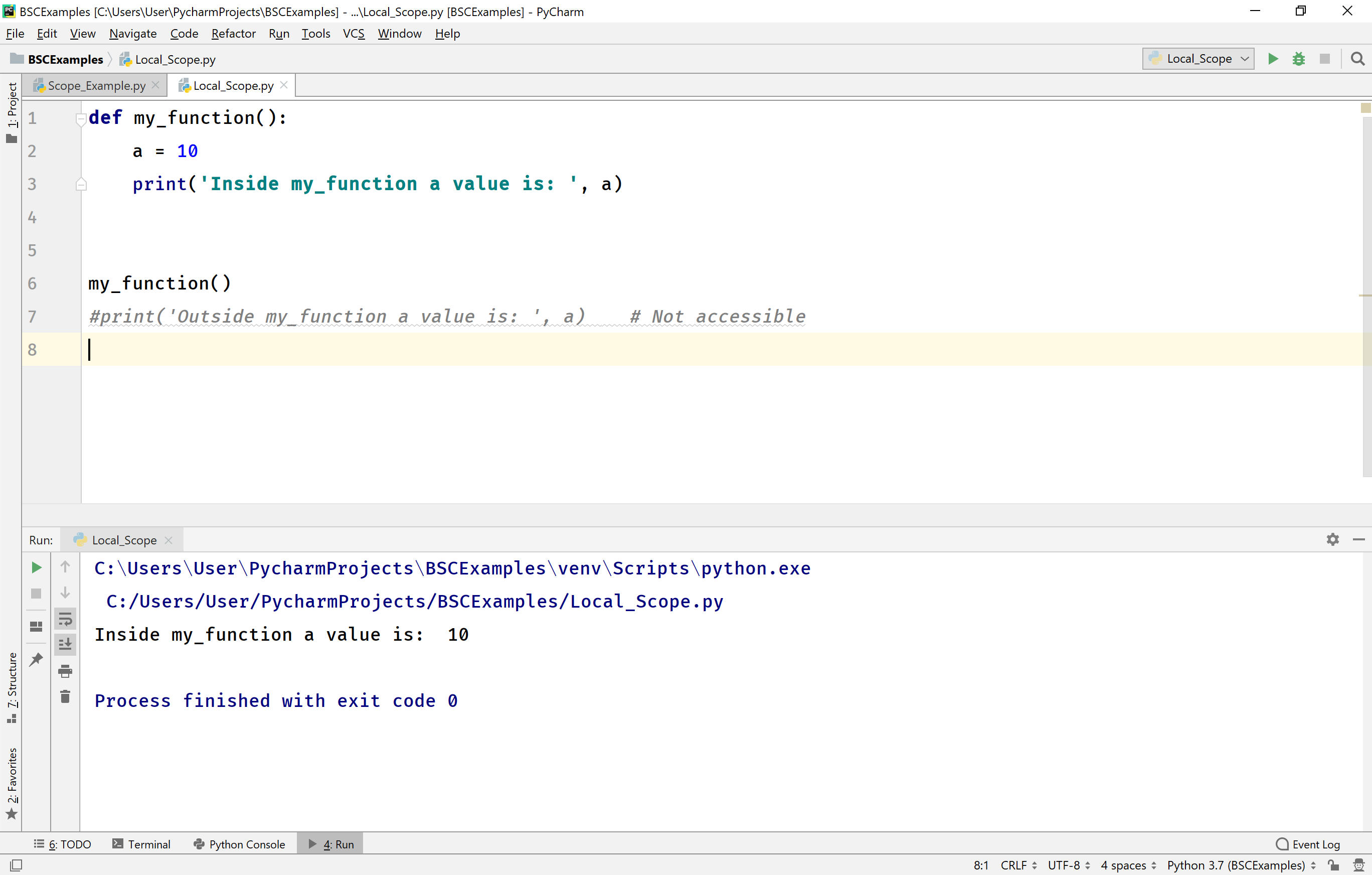When we have a function inside another function, the inner function has access to all variable of both inner and outer functions, but the outer function can access the variables of outer function only it can not access the variables of the inner function. In the following example code, the outer_function has one variable 'a' and the inner_function has one variable 'b'. Here, the inner_function can access both variables 'a' and 'b', but the outer_fucntion can access only variable 'a' but not variable 'b'.

Example
``````def outer_function():
a = 10
def inner_function():
b = 20
print(f'a value inside inner_function is {a}')
print(f'b value inside inner_function is {b}')

print(f'a value inside outer_function is {a}')
inner_function()
#print(f'Trying to access b value from outer_function is {b}')

outer_function()
``````

When we run the above example code, it produces the following output.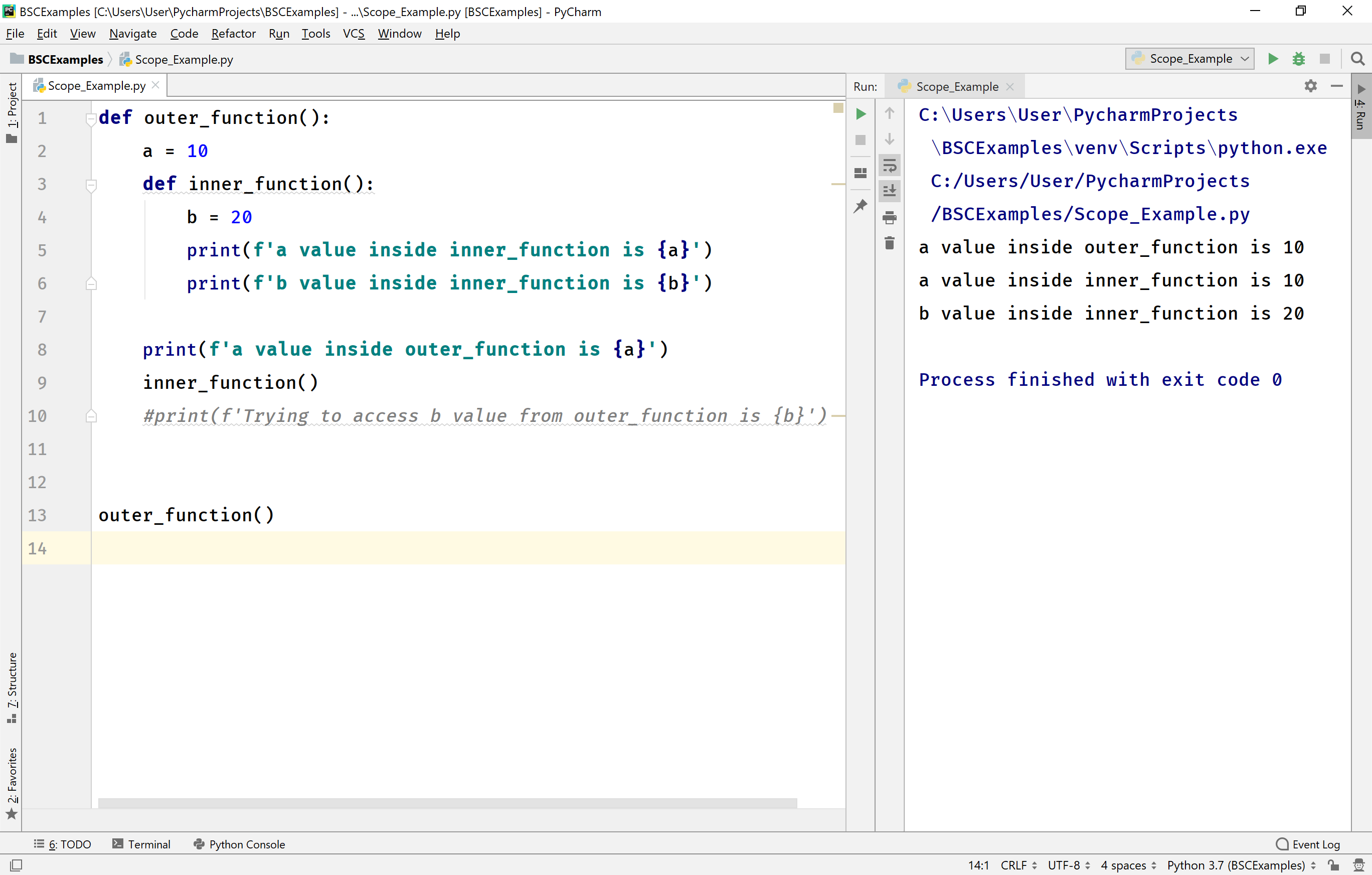# Enclosed Scope

When we have nested functions, the inner function can access the variables of the outer function. The variables of the outer function are called as non-local variables inside the inner function, and they are said to be under the enclosed scope.

We can access the variables of outer function directly inside the inner function. But, we can not modify them directly. To modify those variables, we must write the statement nonlocal variable_name, inside the inner function.

Example
``````def outer_function():
a = 10

def inner_function():
#a = a + 1   # Error- Can't modify
nonlocal a  # Now, can be modified
a = a + 1
print('Inside inner_function a value after modification is: ', a)
inner_function()

outer_function()
``````

When we run the above example code, it produces the following output.# Global Scope

The variable that created before a function is called the global variable, and it is under the global scope. The global variables have access to any function which has defined after the global variable has created.

We can access the global variables directly inside any function in the program. But, we can not modify them directly. To modify those variables, we must write the statement global variable_name, inside the function in which the modification has to be performed.

Example
``````a = 10  # global variable

def my_function_1():
print('Inside my_function_1 a value is: ', a)   #just accessing global variable

def my_function_2():
#a = a + 1 # Error- we can't modify directly
global a    # Now, it can be modified
a = a + 1
print('Inside my_function_2 a value after modification is: ', a)

my_function_1()
my_function_2()

``````

When we run the above example code, it produces the following output.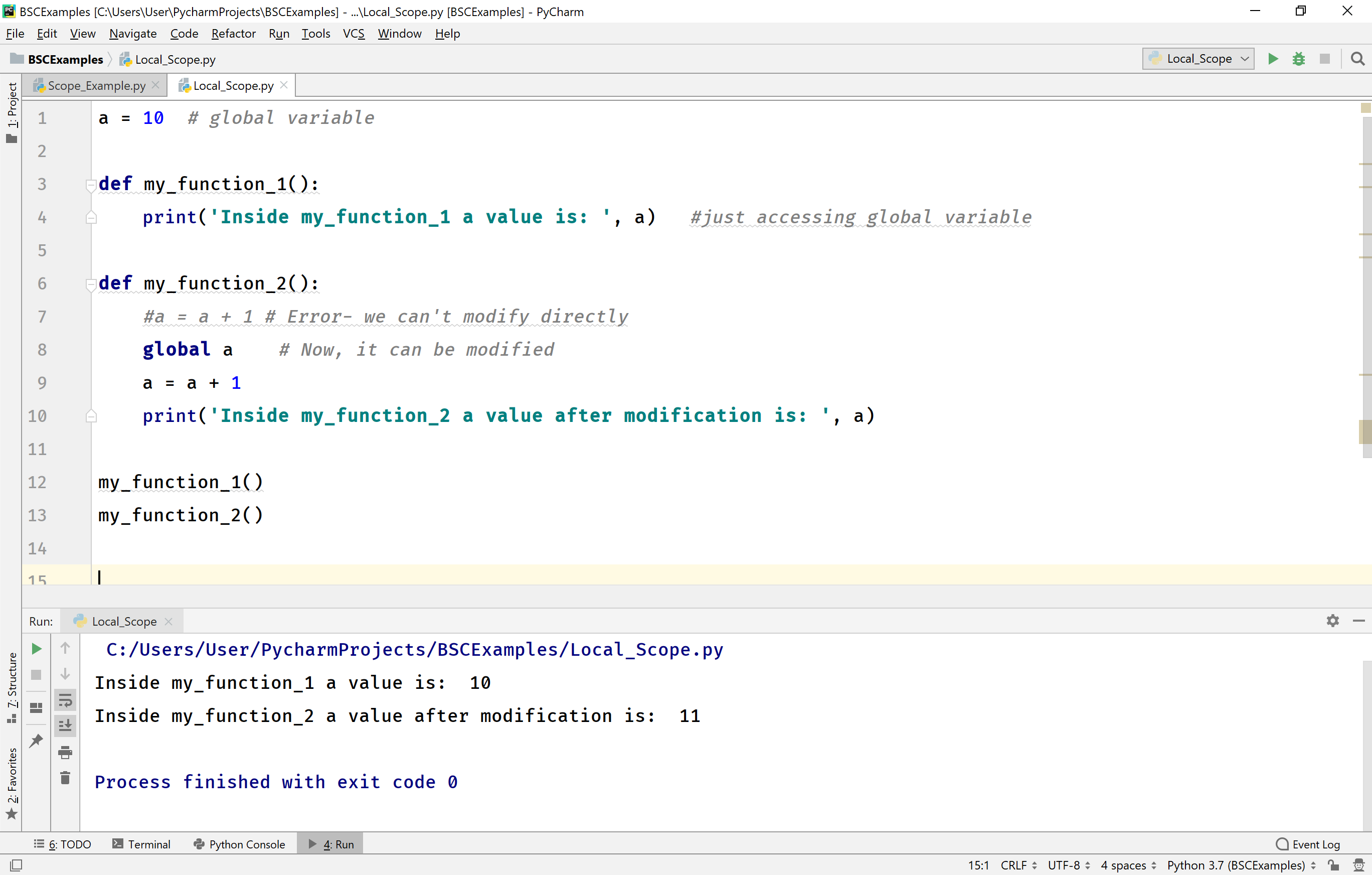# globals() in Python

When we have both a global variable and a local variable with the same name, then the global variable is hidden by the local variable. Inside the function, whenever we use that variable it always refers to the local variable only. In this situation, we use a built-in function globals( ) to access the global variable inside the function.

The built-in function globals() is used to refer the global variables inside a funtion. The general syntax of this funtion is globals( )[ 'global_variable_name' ].

Example
``````a = 10  # global variable

def my_function():
a = 20  # local variable
globals()['a'] = 100
print('Inside my_function, local a value is: ', a)
print('Inside my_function, global a value is: ', globals()['a'])

print('Outside my_function, before calling my_function global variable a value is: ', a)
my_function()
print('Outside my_function, after calling my_function global variable a value is: ', a)
``````

When we run the above example code, it produces the following output.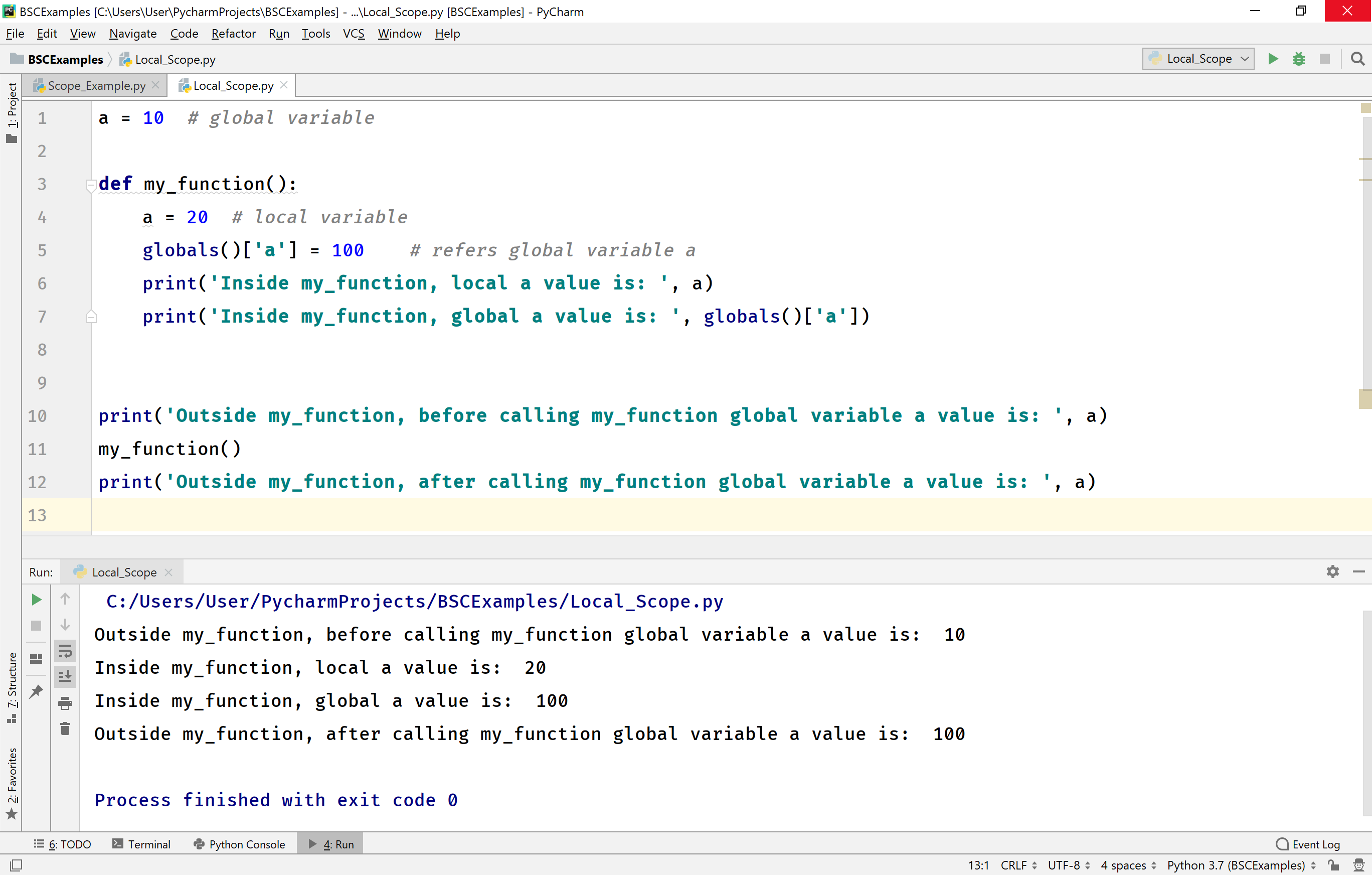# Built-in Scope

The variables and names that are defined in the python built-in modules are said to be under the built-in scope. The names which are under built-in scope can be used directly in the program just by importing the respective module. For example, range( ), print( ), input( ), global, nonlocal, globals( ), and many more.